Prealgebra 2e

# 2.3Solving Equations Using the Subtraction and Addition Properties of Equality

Prealgebra 2e2.3 Solving Equations Using the Subtraction and Addition Properties of Equality

### Learning Objectives

By the end of this section, you will be able to:

• Determine whether a number is a solution of an equation
• Model the Subtraction Property of Equality
• Solve equations using the Subtraction Property of Equality
• Solve equations using the Addition Property of Equality
• Translate word phrases to algebraic equations
• Translate to an equation and solve

### Be Prepared 2.7

Before you get started, take this readiness quiz.

$Evaluatex+8whenx=11.Evaluatex+8whenx=11.$
If you missed this problem, review Example 2.13.

### Be Prepared 2.8

$Evaluate5x−3whenx=9.Evaluate5x−3whenx=9.$
If you missed this problem, review Example 2.14.

### Be Prepared 2.9

Translate into algebra: the difference of $xx$ and $8.8.$
If you missed this problem, review Example 2.24.

When some people hear the word algebra, they think of solving equations. The applications of solving equations are limitless and extend to all careers and fields. In this section, we will begin solving equations. We will start by solving basic equations, and then as we proceed through the course we will build up our skills to cover many different forms of equations.

### Determine Whether a Number is a Solution of an Equation

Solving an equation is like discovering the answer to a puzzle. An algebraic equation states that two algebraic expressions are equal. To solve an equation is to determine the values of the variable that make the equation a true statement. Any number that makes the equation true is called a solution of the equation. It is the answer to the puzzle!

### Solution of an Equation

A solution to an equation is a value of a variable that makes a true statement when substituted into the equation.

The process of finding the solution to an equation is called solving the equation.

To find the solution to an equation means to find the value of the variable that makes the equation true. Can you recognize the solution of $x+2=7?x+2=7?$ If you said $5,5,$ you’re right! We say $55$ is a solution to the equation $x+2=7x+2=7$ because when we substitute $55$ for $xx$ the resulting statement is true.

$x+2=75+2=?77=7✓x+2=75+2=?77=7✓$

Since $5+2=75+2=7$ is a true statement, we know that $55$ is indeed a solution to the equation.

The symbol $=?=?$ asks whether the left side of the equation is equal to the right side. Once we know, we can change to an equal sign $(=)(=)$ or not-equal sign $(≠).(≠).$

### How To

#### Determine whether a number is a solution to an equation.

1. Step 1. Substitute the number for the variable in the equation.
2. Step 2. Simplify the expressions on both sides of the equation.
3. Step 3.
Determine whether the resulting equation is true.
• If it is true, the number is a solution.
• If it is not true, the number is not a solution.

### Example 2.28

$Determine whetherx=5is a solution of6x−17=16.Determine whetherx=5is a solution of6x−17=16.$

### Try It 2.55

$Isx=3a solution of4x−7=16?Isx=3a solution of4x−7=16?$

### Try It 2.56

$Isx=2a solution of6x−2=10?Isx=2a solution of6x−2=10?$

### Example 2.29

$Determine whethery=2is a solution of6y−4=5y−2.Determine whethery=2is a solution of6y−4=5y−2.$

### Try It 2.57

$Isy=3a solution of9y−2=8y+1?Isy=3a solution of9y−2=8y+1?$

### Try It 2.58

$Isy=4a solution of5y−3=3y+5?Isy=4a solution of5y−3=3y+5?$

### Model the Subtraction Property of Equality

We will use a model to help you understand how the process of solving an equation is like solving a puzzle. An envelope represents the variable – since its contents are unknown – and each counter represents one.

Suppose a desk has an imaginary line dividing it in half. We place three counters and an envelope on the left side of desk, and eight counters on the right side of the desk as in Figure 2.3. Both sides of the desk have the same number of counters, but some counters are hidden in the envelope. Can you tell how many counters are in the envelope?

Figure 2.3

What steps are you taking in your mind to figure out how many counters are in the envelope? Perhaps you are thinking “I need to remove the $33$ counters from the left side to get the envelope by itself. Those $33$ counters on the left match with $33$ on the right, so I can take them away from both sides. That leaves five counters on the right, so there must be $55$ counters in the envelope.” Figure 2.4 shows this process.

Figure 2.4

What algebraic equation is modeled by this situation? Each side of the desk represents an expression and the center line takes the place of the equal sign. We will call the contents of the envelope $x,x,$ so the number of counters on the left side of the desk is $x+3.x+3.$ On the right side of the desk are $88$ counters. We are told that $x+3x+3$ is equal to $88$ so our equation is$x+3=8.x+3=8.$

Figure 2.5
$x+3=8x+3=8$

Let’s write algebraically the steps we took to discover how many counters were in the envelope.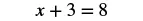First, we took away three from each side.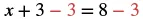Then we were left with five.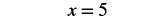Now let’s check our solution. We substitute $55$ for $xx$ in the original equation and see if we get a true statement.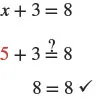Our solution is correct. Five counters in the envelope plus three more equals eight.

### Manipulative Mathematics

Doing the Manipulative Mathematics activity, “Subtraction Property of Equality” will help you develop a better understanding of how to solve equations by using the Subtraction Property of Equality.

### Example 2.30

Write an equation modeled by the envelopes and counters, and then solve the equation: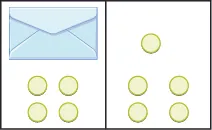### Try It 2.59

Write the equation modeled by the envelopes and counters, and then solve the equation: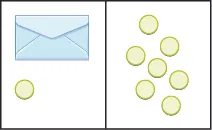### Try It 2.60

Write the equation modeled by the envelopes and counters, and then solve the equation: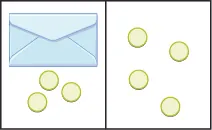### Solve Equations Using the Subtraction Property of Equality

Our puzzle has given us an idea of what we need to do to solve an equation. The goal is to isolate the variable by itself on one side of the equations. In the previous examples, we used the Subtraction Property of Equality, which states that when we subtract the same quantity from both sides of an equation, we still have equality.

### Subtraction Property of Equality

For any numbers $a,b,a,b,$ and $c,c,$ if

$a=ba=b$

then

$a−c=b−ca−c=b−c$

Think about twin brothers Andy and Bobby. They are $1717$ years old. How old was Andy $33$ years ago? He was $33$ years less than $17,17,$ so his age was $17−3,17−3,$ or $14.14.$ What about Bobby’s age $33$ years ago? Of course, he was $1414$ also. Their ages are equal now, and subtracting the same quantity from both of them resulted in equal ages $33$ years ago.

$a=ba−3=b−3a=ba−3=b−3$

### How To

#### Solve an equation using the Subtraction Property of Equality.

1. Step 1. Use the Subtraction Property of Equality to isolate the variable.
2. Step 2. Simplify the expressions on both sides of the equation.
3. Step 3. Check the solution.

### Example 2.31

Solve: $x+8=17.x+8=17.$

### Try It 2.61

Solve:

$x+6=19x+6=19$

### Try It 2.62

Solve:

$x+9=14x+9=14$

### Example 2.32

Solve: $100=y+74.100=y+74.$

### Try It 2.63

Solve:

$95=y+6795=y+67$

### Try It 2.64

Solve:

$91=y+4591=y+45$

### Solve Equations Using the Addition Property of Equality

In all the equations we have solved so far, a number was added to the variable on one side of the equation. We used subtraction to “undo” the addition in order to isolate the variable.

But suppose we have an equation with a number subtracted from the variable, such as $x−5=8.x−5=8.$ We want to isolate the variable, so to “undo” the subtraction we will add the number to both sides.

We use the Addition Property of Equality, which says we can add the same number to both sides of the equation without changing the equality. Notice how it mirrors the Subtraction Property of Equality.

For any numbers $a,ba,b$, and $cc$, if

$a=ba=b$

then

$a+c=b+ca+c=b+c$

Remember the $17-year-old17-year-old$ twins, Andy and Bobby? In ten years, Andy’s age will still equal Bobby’s age. They will both be $27.27.$

$a=ba+10=b+10a=ba+10=b+10$

We can add the same number to both sides and still keep the equality.

### How To

#### Solve an equation using the Addition Property of Equality.

1. Step 1. Use the Addition Property of Equality to isolate the variable.
2. Step 2. Simplify the expressions on both sides of the equation.
3. Step 3. Check the solution.

### Example 2.33

Solve: $x−5=8.x−5=8.$

### Try It 2.65

Solve:

$x−9=13x−9=13$

### Try It 2.66

Solve:

$y−1=3y−1=3$

### Example 2.34

Solve: $27=a−16.27=a−16.$

### Try It 2.67

Solve:

$19=a−1819=a−18$

### Try It 2.68

Solve:

$27=n−1427=n−14$

### Translate Word Phrases to Algebraic Equations

Remember, an equation has an equal sign between two algebraic expressions. So if we have a sentence that tells us that two phrases are equal, we can translate it into an equation. We look for clue words that mean equals. Some words that translate to the equal sign are:

• is equal to
• is the same as
• is
• gives
• was
• will be

It may be helpful to put a box around the equals word(s) in the sentence to help you focus separately on each phrase. Then translate each phrase into an expression, and write them on each side of the equal sign.

We will practice translating word sentences into algebraic equations. Some of the sentences will be basic number facts with no variables to solve for. Some sentences will translate into equations with variables. The focus right now is just to translate the words into algebra.

### Example 2.35

Translate the sentence into an algebraic equation: The sum of $66$ and $99$ is $15.15.$

### Try It 2.69

Translate the sentence into an algebraic equation:

The sum of $77$ and $66$ gives $13.13.$

### Try It 2.70

Translate the sentence into an algebraic equation:

The sum of $88$ and $66$ is $14.14.$

### Example 2.36

Translate the sentence into an algebraic equation: The product of $88$ and $77$ is $56.56.$

### Try It 2.71

Translate the sentence into an algebraic equation:

The product of $66$ and $99$ is $54.54.$

### Try It 2.72

Translate the sentence into an algebraic equation:

The product of $2121$ and $33$ gives $63.63.$

### Example 2.37

Translate the sentence into an algebraic equation: Twice the difference of $xx$ and $33$ gives $18.18.$

### Try It 2.73

Translate the given sentence into an algebraic equation:

Twice the difference of $xx$ and $55$ gives $30.30.$

### Try It 2.74

Translate the given sentence into an algebraic equation:

Twice the difference of $yy$ and $44$ gives $16.16.$

### Translate to an Equation and Solve

Now let’s practice translating sentences into algebraic equations and then solving them. We will solve the equations by using the Subtraction and Addition Properties of Equality.

### Example 2.38

Translate and solve: Three more than $xx$ is equal to $47.47.$

### Try It 2.75

Translate and solve:

Seven more than $xx$ is equal to $37.37.$

### Try It 2.76

Translate and solve:

Eleven more than $yy$ is equal to $28.28.$

### Example 2.39

Translate and solve: The difference of $yy$ and $1414$ is $18.18.$

### Try It 2.77

Translate and solve:

The difference of $zz$ and $1717$ is equal to $37.37.$

### Try It 2.78

Translate and solve:

The difference of $xx$ and $1919$ is equal to $45.45.$

### Section 2.3 Exercises

#### Practice Makes Perfect

Determine Whether a Number is a Solution of an Equation

In the following exercises, determine whether each given value is a solution to the equation.

147.

$x+13=21x+13=21$

1. $x=8x=8$
2. $x=34x=34$
148.

$y+18=25y+18=25$

1. $y=7y=7$
2. $y=43y=43$
149.

$m−4=13m−4=13$

1. $m=9m=9$
2. $m=17m=17$
150.

$n−9=6n−9=6$

1. $n=3n=3$
2. $n=15n=15$
151.

$3p+6=153p+6=15$

1. $p=3p=3$
2. $p=7p=7$
152.

$8q+4=208q+4=20$

1. $q=2q=2$
2. $q=3q=3$
153.

$18d−9=2718d−9=27$

1. $d=1d=1$
2. $d=2d=2$
154.

$24f−12=6024f−12=60$

1. $f=2f=2$
2. $f=3f=3$
155.

$8u−4=4u+408u−4=4u+40$

1. $u=3u=3$
2. $u=11u=11$
156.

$7v−3=4v+367v−3=4v+36$

1. $v=3v=3$
2. $v=11v=11$
157.

$20h−5=15h+3520h−5=15h+35$

1. $h=6h=6$
2. $h=8h=8$
158.

$18 k − 3 = 12 k + 33 18 k − 3 = 12 k + 33$

1. $k=1k=1$
2. $k=6k=6$

Model the Subtraction Property of Equality

In the following exercises, write the equation modeled by the envelopes and counters and then solve using the subtraction property of equality.

159.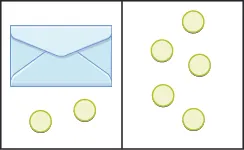160.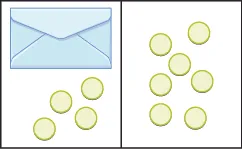161.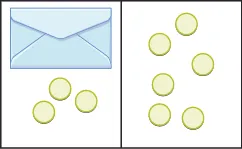162.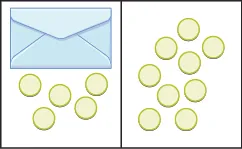Solve Equations using the Subtraction Property of Equality

In the following exercises, solve each equation using the subtraction property of equality.

163.

$a + 2 = 18 a + 2 = 18$

164.

$b + 5 = 13 b + 5 = 13$

165.

$p + 18 = 23 p + 18 = 23$

166.

$q + 14 = 31 q + 14 = 31$

167.

$r + 76 = 100 r + 76 = 100$

168.

$s + 62 = 95 s + 62 = 95$

169.

$16 = x + 9 16 = x + 9$

170.

$17 = y + 6 17 = y + 6$

171.

$93 = p + 24 93 = p + 24$

172.

$116 = q + 79 116 = q + 79$

173.

$465 = d + 398 465 = d + 398$

174.

$932 = c + 641 932 = c + 641$

Solve Equations using the Addition Property of Equality

In the following exercises, solve each equation using the addition property of equality.

175.

$y − 3 = 19 y − 3 = 19$

176.

$x − 4 = 12 x − 4 = 12$

177.

$u − 6 = 24 u − 6 = 24$

178.

$v − 7 = 35 v − 7 = 35$

179.

$f − 55 = 123 f − 55 = 123$

180.

$g − 39 = 117 g − 39 = 117$

181.

$19 = n − 13 19 = n − 13$

182.

$18 = m − 15 18 = m − 15$

183.

$10 = p − 38 10 = p − 38$

184.

$18 = q − 72 18 = q − 72$

185.

$268 = y − 199 268 = y − 199$

186.

$204 = z − 149 204 = z − 149$

Translate Word Phrase to Algebraic Equations

In the following exercises, translate the given sentence into an algebraic equation.

187.

The sum of $88$ and $99$ is equal to $17.17.$

188.

The sum of $77$ and $99$ is equal to $16.16.$

189.

The difference of $2323$ and $1919$ is equal to $4.4.$

190.

The difference of $2929$ and $1212$ is equal to $17.17.$

191.

The product of $33$ and $99$ is equal to $27.27.$

192.

The product of $66$ and $88$ is equal to $48.48.$

193.

The quotient of $5454$ and $66$ is equal to $9.9.$

194.

The quotient of $4242$ and $77$ is equal to $6.6.$

195.

Twice the difference of $nn$ and $1010$ gives $52.52.$

196.

Twice the difference of $mm$ and $1414$ gives $64.64.$

197.

The sum of three times $yy$ and $1010$ is $100.100.$

198.

The sum of eight times $xx$ and $44$ is $68.68.$

Translate to an Equation and Solve

In the following exercises, translate the given sentence into an algebraic equation and then solve it.

199.

Five more than $pp$ is equal to $21.21.$

200.

Nine more than $qq$ is equal to $40.40.$

201.

The sum of $rr$ and $1818$ is $73.73.$

202.

The sum of $ss$ and $1313$ is $68.68.$

203.

The difference of $dd$ and $3030$ is equal to $52.52.$

204.

The difference of $cc$ and $2525$ is equal to $75.75.$

205.

$1212$ less than $uu$ is $89.89.$

206.

$1919$ less than $ww$ is $56.56.$

207.

$325325$ less than $cc$ gives $799.799.$

208.

$299299$ less than $dd$ gives $850.850.$

#### Everyday Math

209.

Insurance Vince’s car insurance has a $500500$ deductible. Find the amount the insurance company will pay, $p,p,$ for an $18001800$ claim by solving the equation $500+p=1800.500+p=1800.$

210.

Insurance Marta’s homeowner’s insurance policy has a $750750$ deductible. The insurance company paid $58005800$ to repair damages caused by a storm. Find the total cost of the storm damage, $d,d,$ by solving the equation $d−750=5800.d−750=5800.$

211.

Sale purchase Arthur bought a suit that was on sale for $120120$ off. He paid $340340$ for the suit. Find the original price, $p,p,$ of the suit by solving the equation $p−120=340.p−120=340.$

212.

Sale purchase Rita bought a sofa that was on sale for $1299.1299.$ She paid a total of $1409,1409,$ including sales tax. Find the amount of the sales tax, $t,t,$ by solving the equation $1299+t=1409.1299+t=1409.$

#### Writing Exercises

213.

Is $x=1x=1$ a solution to the equation $8x−2=16−6x?8x−2=16−6x?$ How do you know?

214.

Write the equation $y−5=21y−5=21$ in words. Then make up a word problem for this equation.

#### Self Check

After completing the exercises, use this checklist to evaluate your mastery of the objectives of this section.

What does this checklist tell you about your mastery of this section? What steps will you take to improve?

Order a print copy

As an Amazon Associate we earn from qualifying purchases.